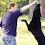## Wednesday, June 12, 2013

### Wednesday Brain Teaser 6-12-13

If you're finding my weekly brain teaser too low stakes, try this one, win a million bucks!

There are 11 ways of expressing the number 100 as a number and fraction using the nine digits once each.
Example:

91+ (5823/647) = 100

The challenge is to find some of the other 10 ways.

Hint: In 9 of them, that first number is above 80.  In one of them, it's less than 10.

1.I can find these two. Took me a while, and I know my method is missing something...

3 + (69258/714) = 100
82 + (3546/197) = 100

1.The full solution set...

100 = 3 + (69258/714)
100 = 81 + (5643/297)
100 = 81 + (7524/396)
100 = 82 + (3546/197)
100 = 91 + (5742/638)
100 = 91 + (5823/647)
100 = 91 + (7524/836)
100 = 94 + (1578/263)
100 = 96 + (1428/357)
100 = 96 + (1752/438)
100 = 96 + (2148/537)

Even though the hints helped reduce the solution space, I still found it easier to spend a couple of hours hammering at computer code than trying to analyze the problem. However, that doesn't mean that some clever thinking would make it easier to find these solutions.